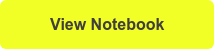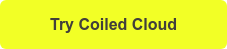# How to parallelize Python code with Dask Delayed

August 30, 2021

Dask Collections are the user interfaces we use for parallel and distributed computing with Dask. Collections can be high-level like Dask DataFrame and Dask Array that provide scalable alternatives to pandas and NumPy respectively; or low-level like Dask Delayed which can be used for general-purpose scalability. Dask Delayed allows us to parallelize custom Python code and algorithms. It can be very powerful in accelerating existing workflows with minimal code changes.

In this post, we will cover:

• How to use Dask Delayed with examples,
• Demonstrate a 10x (or more!) speedup with Dask Delayed, and
• Discuss some best practices along the way.

You can follow along in a notebook when you click below!## General Python Code

Consider the following functions, which perform basic arithmetic operations and sleep for 1 second each:

```def inc(x):
sleep(1)
return x + 1

def dec(x):
sleep(1)
return x - 1

sleep(1)
return x + y```

Calling these functions one-by-one will execute them sequentially. Hence, it will take 3 seconds to compute, and doing this 10 times will correspondingly take 30 seconds:

```l = []

for i in range(10):
a = inc(i)
b = dec(i)
l.append(c)

# takes ~30 seconds to execute```

This computation has great potential for parallelism because:

1. The increment and decrement functions are independent of each other and can be executed in parallel.
2. The for-loop itself can be parallelized, where the 10 iterations are executed in parallel. Figure 1 shows the task graph for this parallel workflow.

We can use Dask Delayed to leverage this potential.

Let’s first spin up a Dask Cluster:

```from dask.distributed import Client, progress
client = Client(n_workers=1)```

Now, you can wrap the functions with the delayed decorator to parallelize them.

```l = []

for i in range(10):
a = delayed(inc)(i)
b = delayed(dec)(i)
l.append(c)

# takes 4.62 ms```

Dask Delayed evaluates lazily, which means the computation is executed only when necessary. Lazy evaluation allows us to work with large datasets in real-time, without waiting for our computations to execute at each step.

Note that Dask Delayed operates on the function and not the result of the function. A common mistake is using `delayed(inc(i))` instead of `delayed(inc)(i)`. The former computes the result before Dask Delayed can help parallelize the function.

At this stage, Dask creates a task graph but doesn’t execute our computation. To visualize the task graph, you can use:

`visualize(*l)`

All tasks in the same row are executed in parallel, therefore the overall computation should take ~3 seconds to complete.

To execute, you can use:

`l = compute(*l)   # takes 3.34 seconds`

This is 10 times faster than our sequential compute and our code is almost the same!

Note that this is collecting all results in the list before calling compute, instead of computing each result in the for loop like: `l.append(c.compute())`. This is a good practice to maximize parallelism.

## Alternative ways to use Dask Delayed

You can create Delayed functions using @delayed before the function definition.

```@delayed
def inc(x):
sleep(1)
return x + 1

@delayed
def dec(x):
sleep(1)
return x - 1

@delayed
sleep(1)
return x + y```

This creates a Delayed function and binds it to the same name, similar to:

```inc = delayed(inc)
dec = delayed(dec)

Now, you can call the functions as usual, and they will operate lazily.

```l = []

for i in range(10):
a = inc(i)
b = dec(i)
l.append(c)

l = compute(*l)

# takes ~3 seconds      ```

## Parallelizing pandas with Dask Delayed

Dask Delayed is versatile and can be also used to parallelize pandas code.

Note that Dask DataFrame is a high-level collection that provides a parallel and distributed alternative for pandas. It is native and optimized for pandas, so we suggest using Dask DataFrame for your workflows. The following example is just for demonstration.

`df = delayed(pd.read_csv)("checkouts-subset.csv")`

This creates a Delayed DataFrame object and makes Delayed objects for all subsequent operations on this DataFrame.